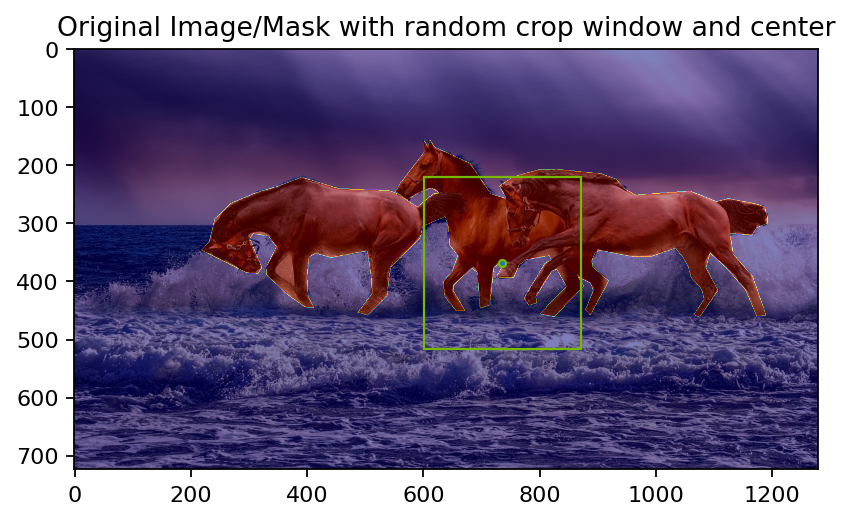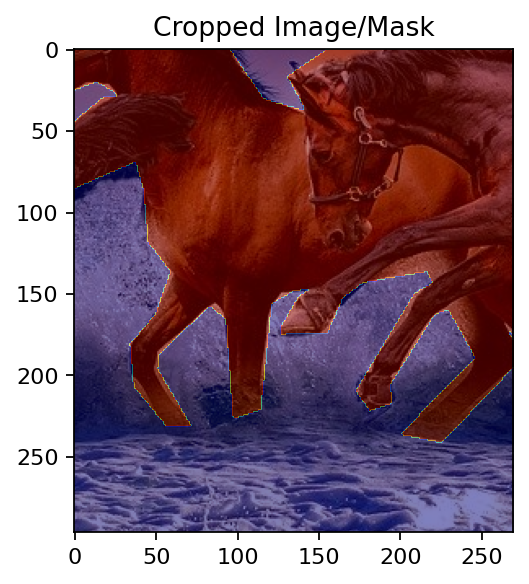Here is an example that demonstrates how to combine the COCO Reader, which loads data from a COCO dataset, with some of the typical augmentations used in image detection and segmentation use cases. The COCO dataset consists of a directory with images and an annotations file containing information about bounding boxes, labels and segmentation masks.

:

from nvidia.dali.pipeline import Pipeline
import nvidia.dali.types as types
import nvidia.dali.fn as fn
import nvidia.dali.math as math
import numpy as np
from time import time
import os.path

import random
random.seed(1231231)   # Random is used to pick colors

test_data_root = os.environ['DALI_EXTRA_PATH']
file_root = os.path.join(test_data_root, 'db', 'coco', 'images')
annotations_file = os.path.join(test_data_root, 'db', 'coco', 'instances.json')

num_gpus = 1     # Single GPU for this example
device_id = 0
batch_size = 32


Let us start by defining a simple pipeline that just loads the data

:

pipe = Pipeline(batch_size=batch_size, num_threads=num_threads, device_id=device_id)
with pipe:
inputs, bboxes, labels, polygons, vertices = fn.readers.coco(
file_root=file_root,
annotations_file=annotations_file,
ltrb=True,          # Bounding boxes to be expressed as left, top, right, bottom coordinates
)
images = fn.decoders.image(inputs, device='mixed')
pipe.set_outputs(images, bboxes, labels, polygons, vertices)
pipe.build()


We can now run the pipeline and visualize the results

:

import matplotlib.pyplot as plt
import matplotlib.patches as patches

H, W = image.shape, image.shape
fig, ax = plt.subplots(dpi=160)

# Displaying the image
ax.imshow(image)

# Bounding boxes
for bbox, label in zip(bboxes, labels):
l, t, r, b = bbox * [W, H, W, H] if relative_coords else bbox
rect = patches.Rectangle((l, t), width=(r - l), height=(b - t),
linewidth=1, edgecolor='#76b900', facecolor='none')

polygon_vertices = mask_vertices[start_vertex:end_vertex]  # Select polygon vertices
# Scale relative coordinates to the image dimensions, if necessary
polygon_vertices = polygon_vertices * [W, H] if relative_coords else polygon_vertices
poly = patches.Polygon(polygon_vertices, True, facecolor='#76b900', alpha=0.7)

plt.show()

def show(outputs, relative_coords=False):
i = 16  # Picked a sample idx that shows more than one bounding box
plot_coco_sample(images.as_cpu().at(i), bboxes.at(i), labels.at(i),
relative_coords=relative_coords)

outputs = pipe.run()
show(outputs)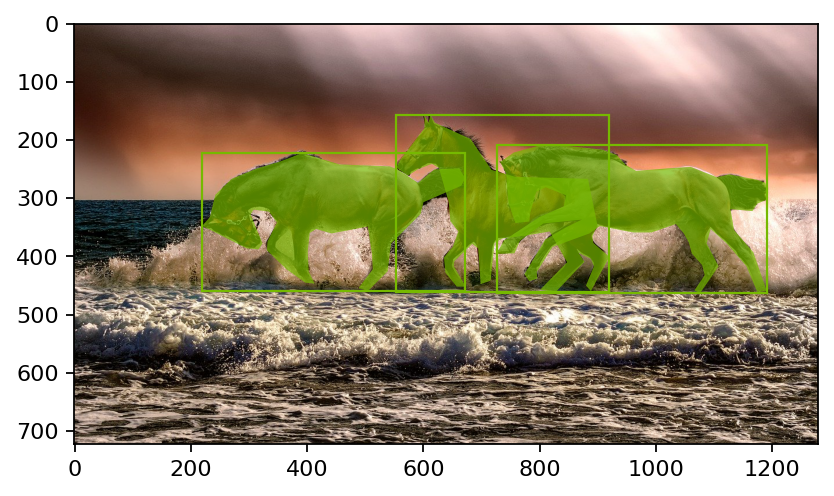A typical augmentation applied in detection and segmentation use cases is a random crop of the image with the restriction that at least one ground truth box is present in the cropped image. In DALI, we use RandomBBoxCrop for that. RandomBBoxCrop operator takes as an input the bounding boxes and the labels associated with them, and a set of constraints for the cropping operation. The result are the cropping window anchor and shape, as well as the processed bounding boxes and labels. The anchor and shape outputs, expressed in relative coordinates, can be directly fed into DALI’s Slice operator to extract the region of interest of the image. The output bounding boxes and labels are processed to contain only the ones within the cropping window, and the coordinates are mapped to the new coordinate space. RandomBBoxCrop does not process segmentation masks, so the mask coordinates need to be mapped to the new coordinate space separately.

:

# Wrapping the pipeline definition in separate functions that we can reuse later

inputs, bboxes, labels, polygons, vertices = fn.readers.coco(
file_root=file_root,
annotations_file=annotations_file,
ratio=True,         # Bounding box and mask polygons to be expressed in relative coordinates
ltrb=True,          # Bounding boxes to be expressed as left, top, right, bottom coordinates
)
return inputs, bboxes, labels, polygons, vertices

def random_bbox_crop_def(bboxes, labels, polygons, vertices):
# RandomBBoxCrop works with relative coordinates
# The arguments have been selected to produce a significantly visible crop
# To learn about all the available options, see the documentation
anchor_rel, shape_rel, bboxes, labels, bbox_indices = fn.random_bbox_crop(
bboxes,
labels,
aspect_ratio=[0.5, 2],     # Range of aspect ratios
thresholds=[0.0],          # No minimum intersection-over-union, for demo purposes
allow_no_crop=False,       # No-crop is disallowed, for demo purposes
scaling=[0.3, 0.6],        # Scale range of the crop with respect to the image shape
seed=12345,                # Fixed random seed for deterministic results
bbox_layout="xyXY",        # left, top, right, back
output_bbox_indices=True,  # Output indices of the filtered bounding boxes
)

# Select mask polygons of those bounding boxes that remained in the image
bbox_indices, polygons, vertices
)

return anchor_rel, shape_rel, bboxes, labels, polygons, vertices

with pipe:
inputs, bboxes, labels, polygons, vertices = coco_reader_def()
anchor_rel, shape_rel, bboxes, labels, polygons, vertices = \
random_bbox_crop_def(bboxes, labels, polygons, vertices)

# Partial decoding of the image
images = fn.decoders.image_slice(
inputs, anchor_rel, shape_rel, normalized_anchor=True, normalized_shape=True, device='cpu'
)
# Cropped image dimensions
crop_shape = fn.shapes(images, dtype=types.FLOAT)
crop_h = fn.slice(crop_shape, 0, 1, axes=)
crop_w = fn.slice(crop_shape, 1, 1, axes=)

images = images.gpu()

# Adjust masks coordinates to the coordinate space of the cropped image, while also converting
# relative to absolute coordinates by mapping the top-left corner (anchor_rel_x, anchor_rel_y), to (0, 0)
# and the bottom-right corner (anchor_rel_x+shape_rel_x, anchor_rel_y+shape_rel_y) to (crop_w, crop_h)
MT_vertices = fn.transforms.crop(
from_start=anchor_rel, from_end=(anchor_rel + shape_rel),
to_start=(0.0, 0.0), to_end=fn.cat(crop_w, crop_h)
)
vertices = fn.coord_transform(vertices, MT=MT_vertices)

# Convert bounding boxes to absolute coordinates
MT_bboxes = fn.transforms.crop(
to_start=(0.0, 0.0, 0.0, 0.0), to_end=fn.cat(crop_w, crop_h, crop_w, crop_h)
)
bboxes = fn.coord_transform(bboxes, MT=MT_bboxes)

pipe.set_outputs(images, bboxes, labels, polygons, vertices)

pipe.build()
outputs = pipe.run()
show(outputs)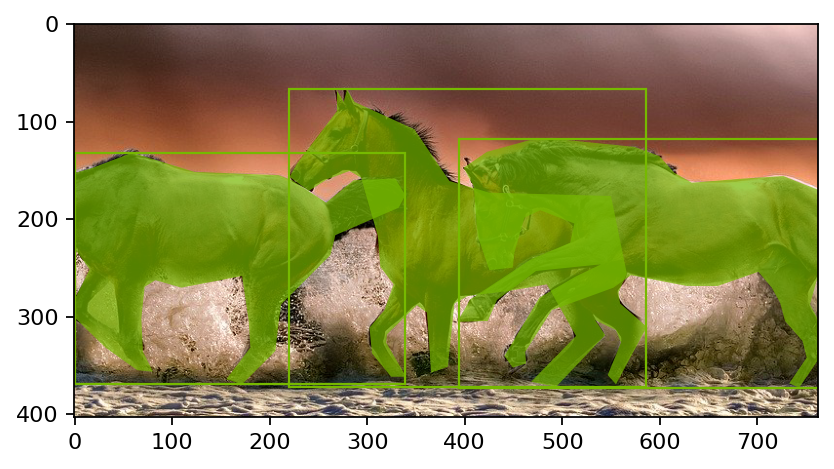In the following pipeline, we paste the image to a larger canvas and flip it horizontally

:

pipe = Pipeline(batch_size=batch_size, num_threads=num_threads, device_id=device_id, seed=12345)
with pipe:
inputs, bboxes, labels, polygons, vertices = coco_reader_def()
orig_shape = fn.peek_image_shape(inputs)
images = fn.decoders.image(inputs)
images = images.gpu()
px = fn.random.uniform(range=(0, 1))
py = fn.random.uniform(range=(0, 1))
ratio = fn.random.uniform(range=(1, 2))
images = fn.paste(images, paste_x=px, paste_y=py, ratio=ratio, fill_value=(32, 64, 128))
bboxes = fn.bbox_paste(bboxes, paste_x=px, paste_y=py, ratio=ratio, ltrb=True)

scale = 1.0 / ratio
margin = ratio - 1.0
px_1 = scale * px * margin
py_1 = scale * py * margin
ver_x = scale * fn.slice(vertices, 0, 1, axes=) + px_1
ver_y = scale * fn.slice(vertices, 1, 1, axes=) + py_1
vertices = fn.cat(ver_x, ver_y, axis=1)

should_flip = fn.random.coin_flip(probability=1.0)  # 100% probability for demo purposes
images = fn.flip(images, horizontal=should_flip)
bboxes = fn.bb_flip(bboxes, horizontal=should_flip, ltrb=True)
vertices = fn.coord_flip(vertices, flip_x=should_flip)

pipe.set_outputs(images, bboxes, labels, polygons, vertices)

pipe.build()
outputs = pipe.run()
show(outputs, relative_coords=True)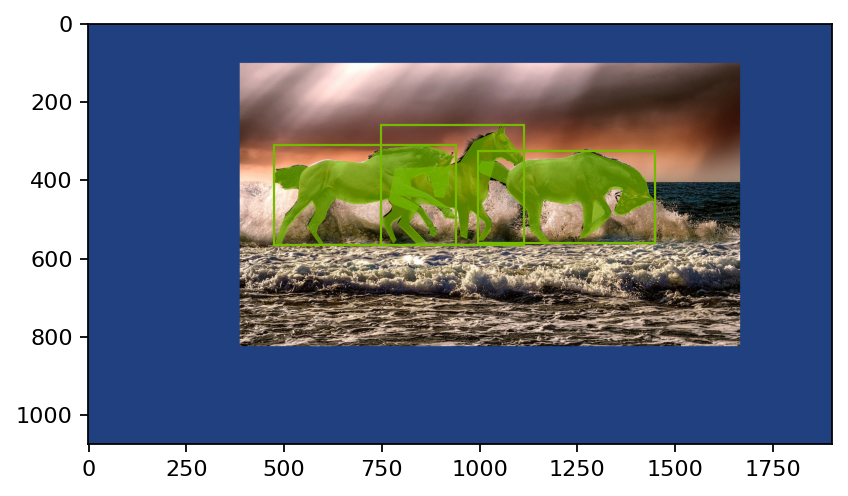In some segmentation and detection datasets, the density of objects is very low. This can cause the background to be overrepresented during training when randomly cropping the image. To compensate for that, we can select crop windows to be centered at foreground pixels with a certain probability.

:

pipe = Pipeline(batch_size=batch_size, num_threads=num_threads, device_id=device_id, seed=11)
with pipe:
file_root=file_root,
annotations_file=annotations_file,
)
images = fn.decoders.image(inputs)

# COCO reader produces three dimensions (H, W, 1). Here we are just removing the trailing dimension
# rel_shape=(1, 1) means keep the first two dimensions as they are.

# Select random foreground pixels with 70% probability and random pixels with 30% probability
# Foreground pixels are by default those with value higher than 0.
)

# Random crop shape (can also be constant)
crop_h = fn.cast(fn.random.uniform(range=(200, 300), shape=(1,), device='cpu'), dtype=types.INT64)
crop_w = fn.cast(fn.random.uniform(range=(200, 300), shape=(1,), device='cpu'), dtype=types.INT64)
crop_shape = fn.cat(crop_h, crop_w, axis=0)

# Calculating anchor for slice (top-left corner of the cropping window)
crop_anchor = center - crop_shape // 2

# Note that we are allowing padding when sampling out of bounds, since a foreground pixel can appear
# near the edge of the image.
out_image = fn.slice(images, crop_anchor, crop_shape, axis_names="HW", out_of_bounds_policy='pad')

pipe.build()
outputs = pipe.run()
i = 16
image = outputs.at(i)
center = outputs.at(i)
anchor = outputs.at(i)
shape = outputs.at(i)
out_image = outputs.at(i)

fig, ax = plt.subplots(dpi=160)
ax.imshow(image)
rect = patches.Rectangle((anchor, anchor), width=shape, height=shape,
linewidth=1, edgecolor='#76b900', facecolor='none')